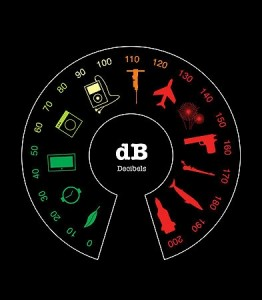# Adding decibels while Mixing Tracks

## Decibels | Introduction

A lot of people ask questions about adding decibels. Doubts are quite reasonable and they will start showing up as soon as we start thinking about the problems.

For example, the sound pressure level of a simple silent 12 cm fan in your compute is 21 dB.

Does it mean that the total sound pressure of 7 silent computer fans will get as high as 147 dB and make us deaf. Well, every sane person will answer: of course No. Why is it so? And how we should actually add decibels, that’s what I will try to explain in this very short post.

So.Decibels (we are speaking of dB SPL). We can add them the basic way, we are used to, because, as we know it, it is based on the logarithmic scale.

Which means, that 60 dB + 60 dB won’t give us 120 dB.

Adding decibels is way too complicated to do it without logarithms. I created an easy calculator, that will help us do that.

The calculator is based on a model, that will help you sum the decibels.

##Where:

Li – i level pressure (in dB)

n – number of sources

Warning!

The model should be used only for summing different sound sources. It’s not useful for summing identical levels or similar signals, as it will not take phase dependencies and wave coherence into account.

The results may surprise you, but that’s how decibels are added.

For the sake of convenience, I will mention 2 simple rules, that can be applied, and will certainly help you add your decibels quite fast and approximate.

• When adding the same value.

## The Maths of decibel adding

The sum of two same values gives us the value +3db.

e.g.

24 dB + 24 dB = 27dB

100  dB + 100 dB = 103 dB

• Adding two values, which difference is more than 10

• The sum of two levels, that vary by more than 10

e.g.

10 dB + 60 dB = 60 dB

40 dB + 60 dB = 60 dB

It may look quite weird, but it’s the way it works

At the end, i’m going to show how it looks like when we add additional audio sources of the same level. Let’s say someone is playing the guitar: the sound pressure level is 80 db, if another musicians joined playing on another instrument but with the same SPL level, the total would be only 83 dB (we learnt that with the first rule). If a third were to join, he would add only 1,78 db to the total SPL.

So our three guys would generate the sound pressure level of: 80+3+1,78 = 84,8 dB. With each musician we would gain less and less.

If we wanted to get a gain of 3 dB, we’d have to have 1000 people playing!

Table. Increase in the sound pressure level, when adding new sources.

As you can clearly see, if keep adding sources after the fourth, the total raise of the SP level will be less that 1 dB, which basically means we won’t hear the difference in sound, when the 5th source with the same SPL will join. (A human can hear the change in acoustic pressure(acoustic loops), if it is higher than 1dB)

I hope i put a little light on the problem, and answered the question on adding decibels. I advise you to use the calculator as well.

Check out another great blog.